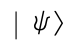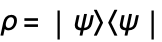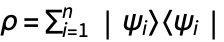# The Density Matrix and the Liouville Equation

The density matrix is a generalization of the state vector that allows graceful handling of ensemble averages and relaxation effects. For a single atom with state vector, we define the density operator by.

 Define an atomic system and form its ket vector.
 Taking the outer product of the ket with its dual gives the density matrix in terms of the state vector coefficients.

Evidently, the diagonal matrix elements of the density operator are the probabilities of the corresponding states (here the states 1 and 2). The off-diagonal matrix elements are known as coherences.

We can retrace the example given in "The State Vector and the Schrödinger Equation" in terms of the density-matrix elements rather than the state-vector amplitudes. The equation governing the evolution of the density matrix,, known as the Liouville equation, can be derived directly from the Schrödinger equation.

 The function DensityMatrix forms the density matrix for an atomic system.
 The Hamiltonian describing the interaction with an electric field. We normalize the electric field to frequency units by dividing by the reduced dipole matrix element between states 1 and 2.
 The Liouville equation.
 Initial conditions, specifying that the atom is in state 1 at time zero.
 List of the density-matrix variables.
 Solve the Liouville equation.
 The diagonal density-matrix elements are the probabilities of finding each state.

If instead of a single atom, we are interested in averages over an ensemble ofatoms, each with state vector |ψi, we simply work with the averaged density matrix:.